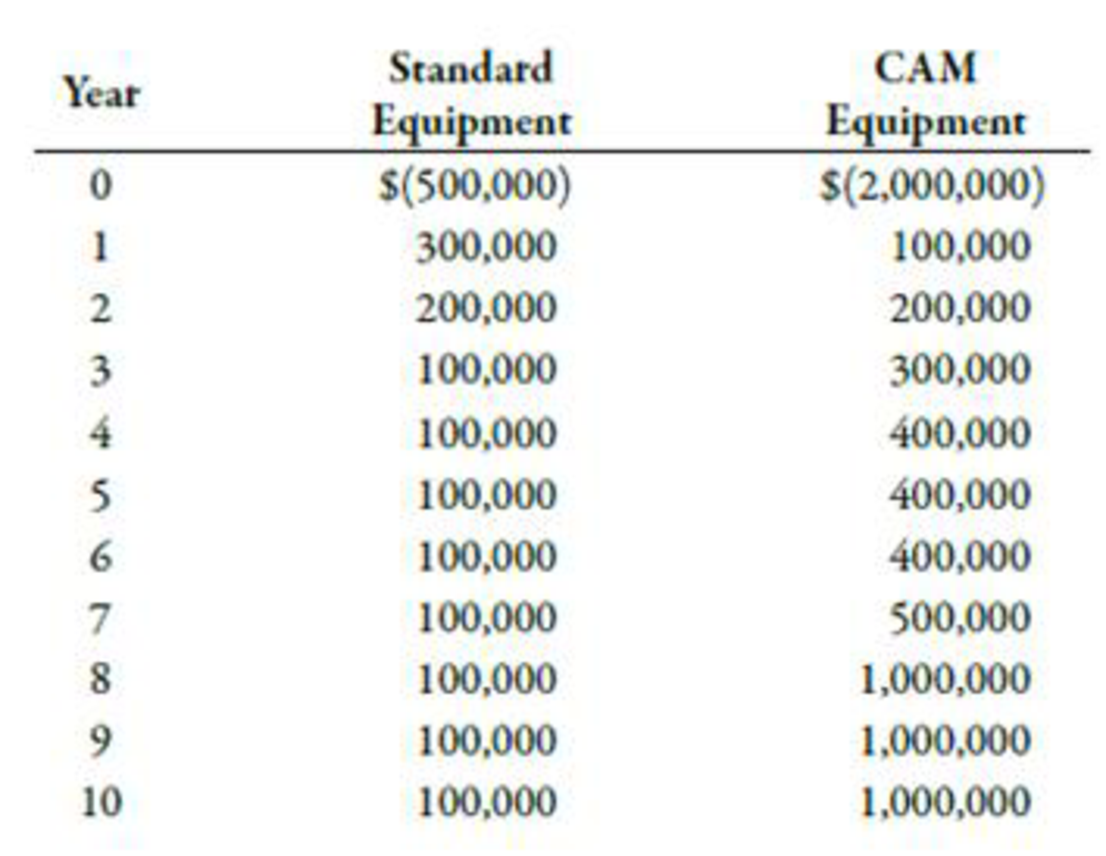Chapter 12, Problem 52P### Managerial Accounting: The Corners...

7th Edition
Maryanne M. Mowen + 2 others
ISBN: 9781337115773

#### Solutions

Chapter
Section### Managerial Accounting: The Corners...

7th Edition
Maryanne M. Mowen + 2 others
ISBN: 9781337115773
Textbook Problem
41 views

# Patterson Company is considering two competing investments. The first is for a standard piece of production equipment. The second is for computer-aided manufacturing (CAM) equipment. The investment and after-tax operating cash flows follow:Patterson uses a discount rate of 18% for all of its investments. Patterson’s cost of capital is 10%.Required: 1. Calculate the NPV for each investment by using a discount rate of 18%. 2. Calculate the NPV for each investment by using a discount rate of 10%. 3. CONCEPTUAL CONNECTION Which rate should Patterson use to compute the NPV? Explain

1.

To determine

Find out the NPV of each method by using a discount rate of 18%.

Explanation

Net Present Value:

The remaining balance of the present value of a project’s inflows and outflows is known as net present value (NPV). It is a discounting model of capital investment decision. A project with a positive NPV increases the wealth of a firm whereas a project with a negative NPV decreases the wealth of a firm.

Calculate NPV for standard equipment:

2.

To determine

Find out the NPV of each method by using a discount rate of 10%.

3.

To determine

Discuss the discount rate to be used in calculation of NPV.

### Still sussing out bartleby?

Check out a sample textbook solution.

See a sample solution

#### The Solution to Your Study Problems

Bartleby provides explanations to thousands of textbook problems written by our experts, many with advanced degrees!

Get Started

#### Why is productivity important?

Essentials of Economics (MindTap Course List)

#### Accounts Payable is an example of an asset account.

College Accounting, Chapters 1-27 (New in Accounting from Heintz and Parry)

#### Finally, you can also use the information on the Internet to value the entire corporation. This approach requir...

Fundamentals of Financial Management, Concise Edition (with Thomson ONE - Business School Edition, 1 term (6 months) Printed Access Card) (MindTap Course List)